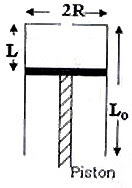## Tuesday, May 15, 2007

### IIT-JEE 2007 Linked Comprehension type Questions (MCQ) on Properties of Matter

Among the IIT-JEE 2007 physics questions there were 4 Linked Comprehension Type questions, each paper carrying two of them. Each Linked Comprehension Type question contained a paragraph based on which three multiple choice questions were asked. Here is the question on properties of matter:

Paragraph for the multiple choice questions:A fixed thermally conducting cylinder has a radius R and height L0. The cylinder is open at the bottom and has a small hole at its top. A piston of mass M is held at a distance L from the top surface, as shown in the figure. The atmospheric pressure is P0.

Questions:

(1) The piston is now pulled out slowly and held at a distance 2L from the top. The pressure in the cylinder between its top and the piston will then be

(A) P0 (B) P0/2 (C) P0/2 + Mg/πR2 (D) P0/2 Mg/πR2

The correct option is (A) since the air will enter the cylinder through the hole at the top and will keep the pressure at P

(2) While the piston is at a distance 2L from the top, the hole at the top is sealed. The piston is then released, to a position where it can stay in equilibrium. In this condition, the distance of the piston from the top is

(A)[2P0πR2/(πR2P0 +Mg)](2L)

(B) [(P0πR2–Mg)/πR2P0](2L)

(C)[(P0πR2 +Mg)/πR2P0](2L)

(D) [P0πR2/( πR2P0 –Mg)](2L)

If P is the pressure inside (the region between the piston and the top of the cylinder), the weight of the piston is balanced by the thrust (P0 – P)πR2 arising due to the pressure difference between the top and the bottom of the cylinder. Therefore,

(P0 P)πR2 = Mg from which P = P0 –Mg/πR2 = (πR2P0 Mg)/πR2

Now, substitute this value of P in the relation, P0×2L = P×L’, where L’ is the final distance of the piston from the top. [Note that this equation follows from Boyle’s law].

Thus, P0×2L = [(πR2P0 Mg)/ πR2] × L' from which

L' = [P0πR2/( πR2P0 Mg)](2L).

(3) The piston is taken completely out of the cylinder. The hole at the top is sealed. A water tank is brought below the cylinder and put in a position so that the water surface in the tank is at the same level as the top of the cylinder as shown in the figure. The density of water is ρ. In equilibrium, the height H of the water column in the cylinder satisfies(A) ρg(L0–H)2 +P0(L0–H)+ L0P0 = 0

(B) ρg(L0–H)2 –P0(L0–H)– L0P0 = 0

(C) ρg(L0–H)2 +P0(L0–H)– L0P0 = 0

(D) ρg(L0–H)2 –P0(L0–H)+ L0P0 = 0

The correct option is obtained by applying Boyle’s law. The initial pressure of air column of length L0 is the atmospheric pressure P0. The final pressure of the air column of length (L0–H) is [(P0+(L0–H)ρg] since the pressure of air inside the cylinder exceeds the atmospheric pressure by the hydrostatic pressure exerted by water column of height (L0–H).

Applying Boyle's law, P0L0 = [(P0 +(L0–H)ρg](L0–H).

Rearranging this you will get the equation in option (c).

#### 1 comment:

1.Anonymous5:37 PM

Howdy! Someone in my Myspace group shared this website with us so I came
to take a look. I'm definitely enjoying the information.

I'm bookmarking and will be tweeting this to my
followers! Terrific blog and superb design and style.

Stop by my homepage vexxhost product reviews ()